## Example Questions

### Example Question #1 : How To Find An Exponent From A Rational Number

Which real number satisfies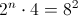?

Possible Answers:Correct answer:Explanation:

Simplying the equation, we get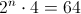.

This further simplifies to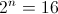.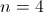satisfies this equation. You could also use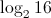to determine that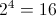.

### Example Question #2 : How To Find An Exponent From A Rational Number

Find the value ofif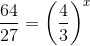Possible Answers:Correct answer:Explanation:

When a fraction is raised to an exponent, both the numerator and denominator are raised to that exponent. Therefore, the equation can be rewritten as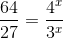. From here we can proceed one of two ways. We can either solve x for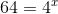or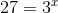. Let's solve the first equation. We simply multiply 4 by itself until we reach a value of 64.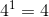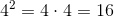,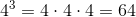, and so on. Since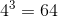, we know that x = 3.

We can repeat this process for the second equation to get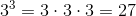, confirming our previous answer. However, since the ACT is a timed test, it is best to only solve one of the equations and move on. Then, if you have time left once all of the questions have been answered, you can come back and double check your answer by solving the other equation.

### Example Question #3 : How To Find An Exponent From A Rational Number

If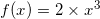and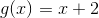, what is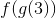?

Possible Answers: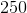Correct answer:Explanation:

Start from the inside.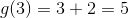. Then,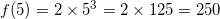.

### Example Question #4 : How To Find An Exponent From A Rational Number

Simplify by expressing each term in exponential form.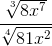Possible Answers: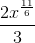None of these are correct.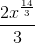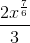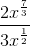Correct answer:Explanation:

The rule for exponential ratios is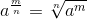.

Using this, we can convert the numerator and denominator quickly.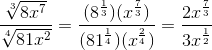The middle step in the coversion is important, as there is a big difference between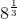and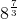, and likewise for the denominator.

Next, we can further simplify by remembering that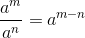Find the least common denominator and simplify: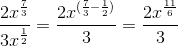Thus,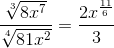### Example Question #5 : How To Find An Exponent From A Rational Number

Often, solving a root equation is as simple as switching to exponential form.

Simplify into exponential form: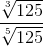Possible Answers: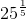None of these are correct.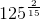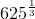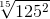Correct answer:Explanation:

The rule for exponential ratios is.

Using this, we can convert the numerator and denominator quickly.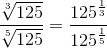Next, we can further simplify by remembering thatFind the least common denominator and simplify: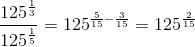Thus, our answer is. (Remember, the problem asked for exponential form!)

### All ACT Math Resources